# OASP - 2

## 傅里叶变换

Posted by mingjie on November 11, 2017

### 定义

$\sigma$$x = 0$的时候，傅里叶和逆傅里叶变换变为

### 常见的傅里叶变换

##### 方波##### 高斯函数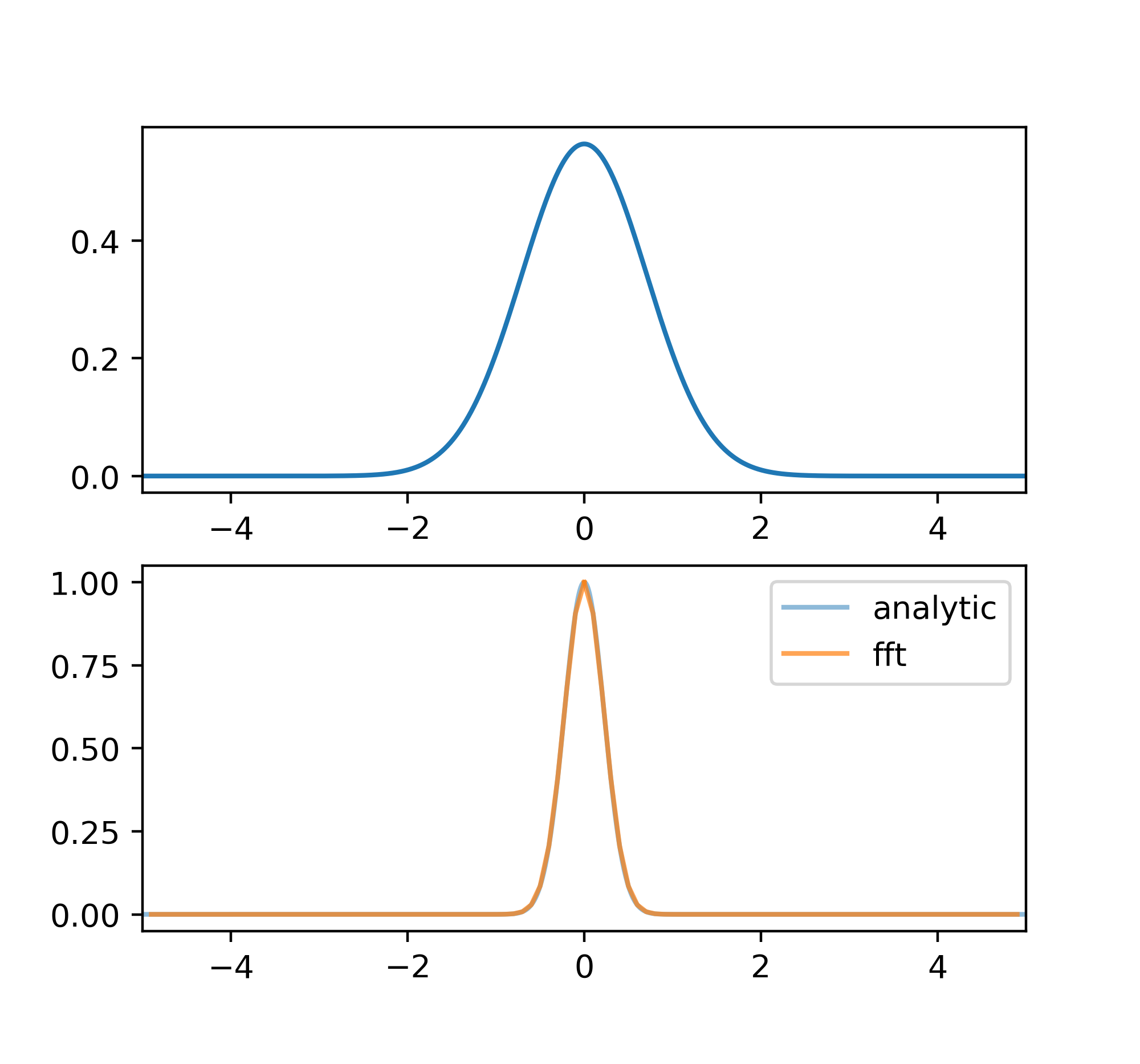##### 洛仑兹函数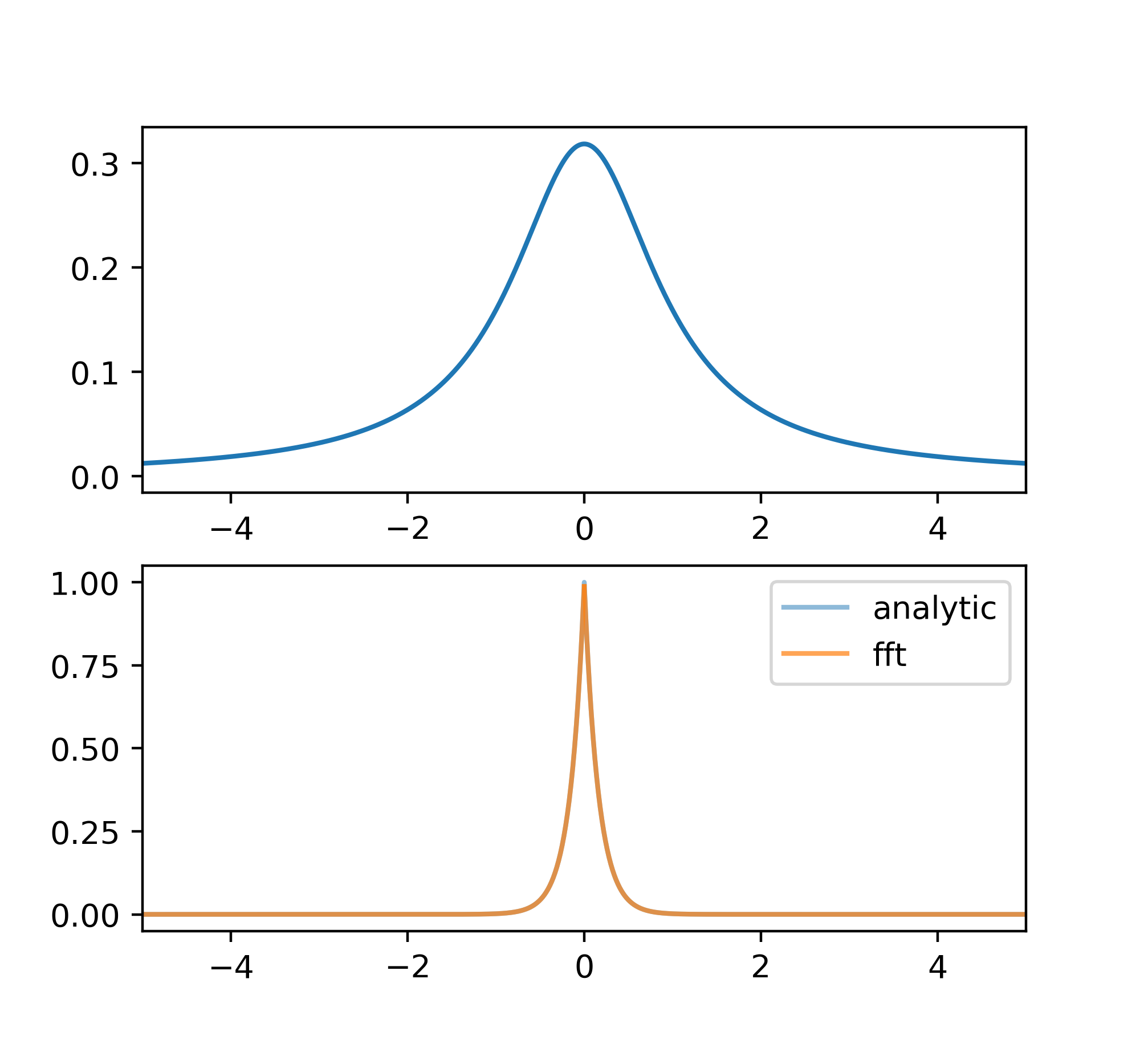##### $\delta$函数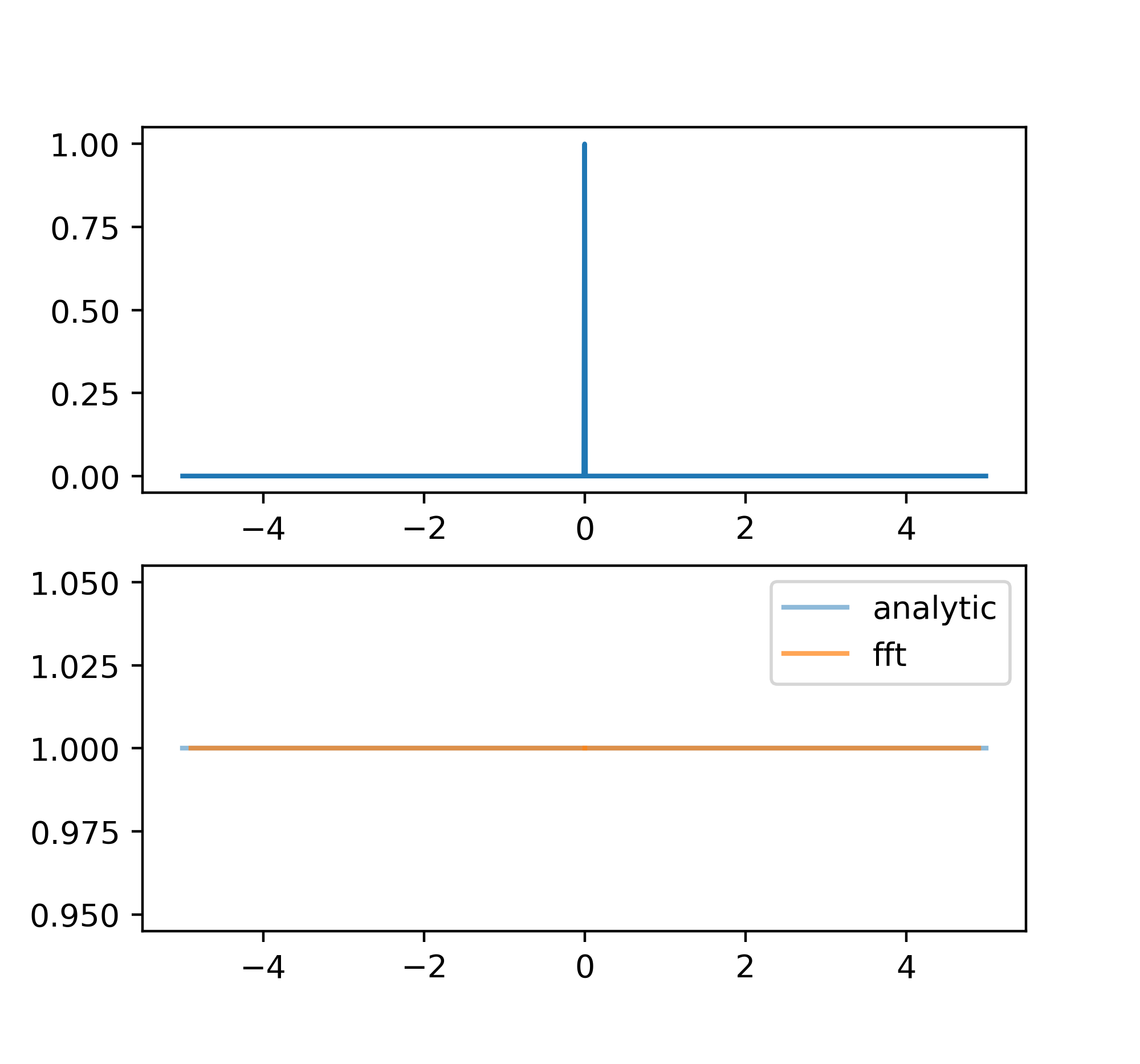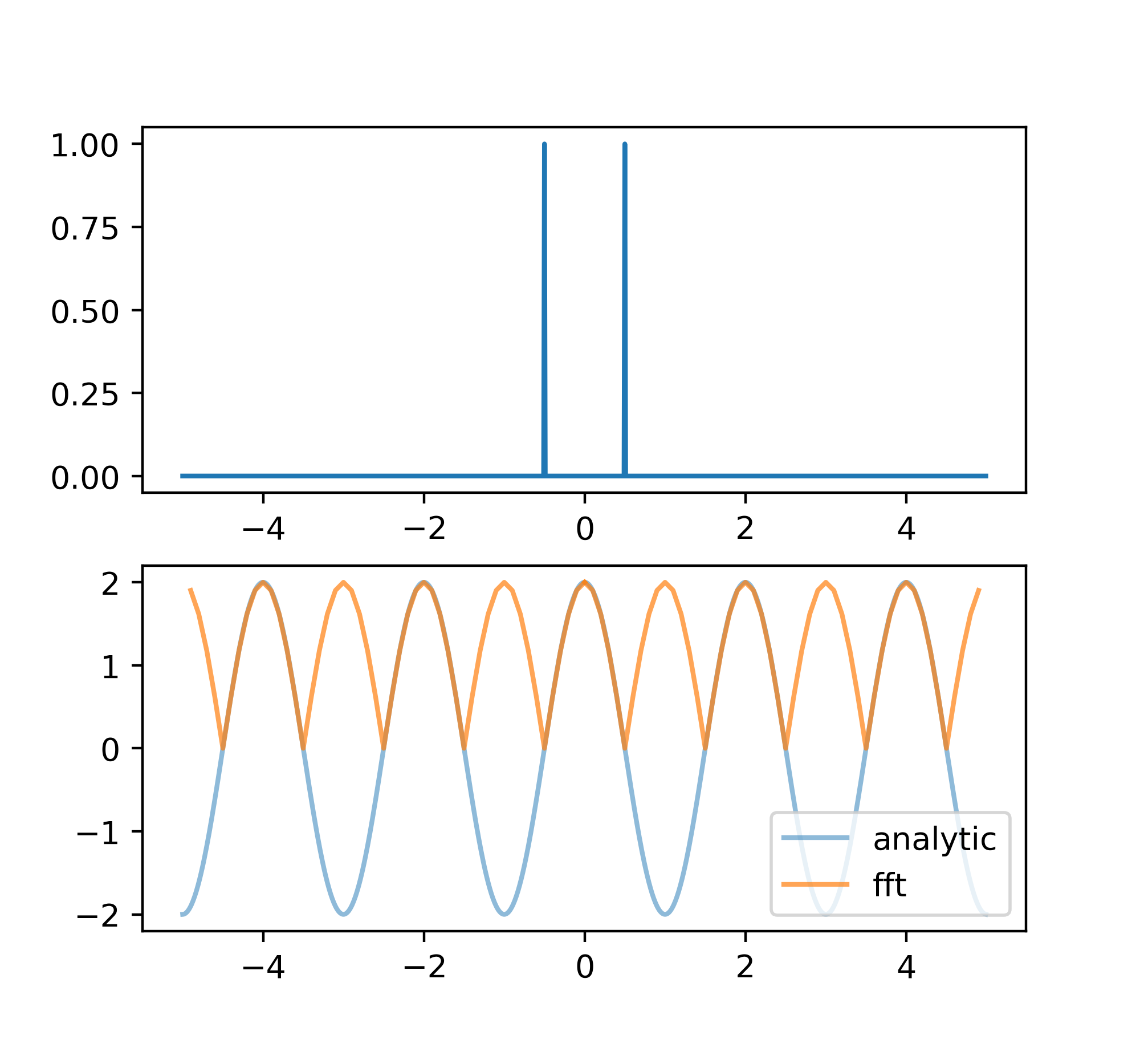##### Shah函数

Shah函数实际上是一堆等间距分布的$\delta$函数：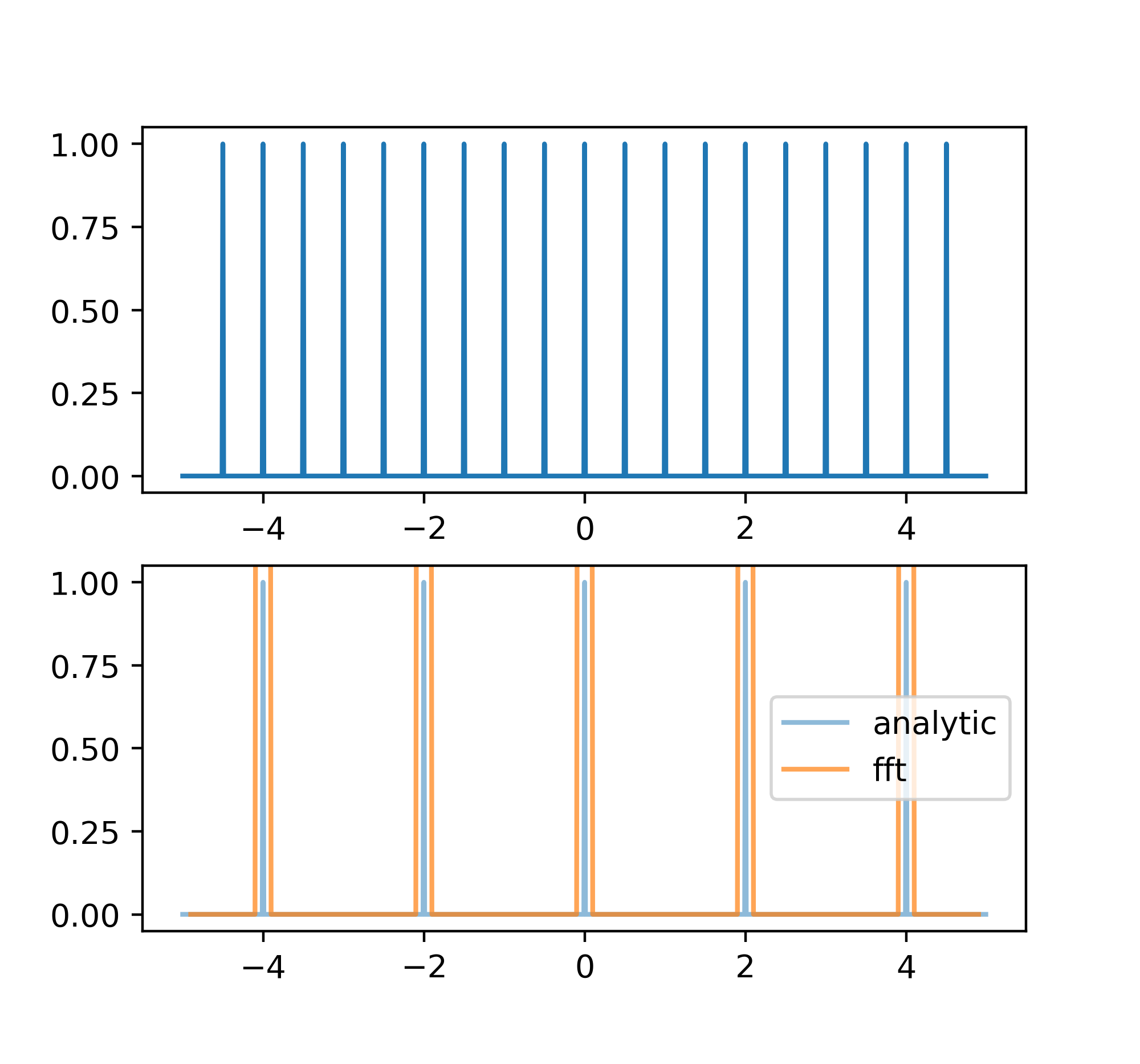### 卷积### 被模糊的数据

$\sigma$域，上式变为

### 一些定理

1. 定义$z = \frac{\int xF(x) \mathrm{d}x}{\int F(x) \mathrm{d}x}$，则若$K(x) = F(x) * G(x)$，有$z_K = z_F + z_G$

### 离散计算

FFT中的输入和结果都是离散的，也就是说$D(x)$变成了$D(j\Delta x)$$j$是整数，从$0$$N$。同理，将结果也离散化成$\sigma = k \Delta \sigma$，从傅里叶变换的定义出发我们可以得到

##### 有关numpy中的fft

（暂未解决shah函数的fft结果和预计不符的问题）

### 误差

（实际上我们并不一定能知道$E(x)$，更多时候是知道$E(x)$的一些性质比如说平均值，这个时候怎么办？）

（书里提到了FFT的数组长度必须为2的指数，但是实际上似乎不是？）

### 时间序列（略）